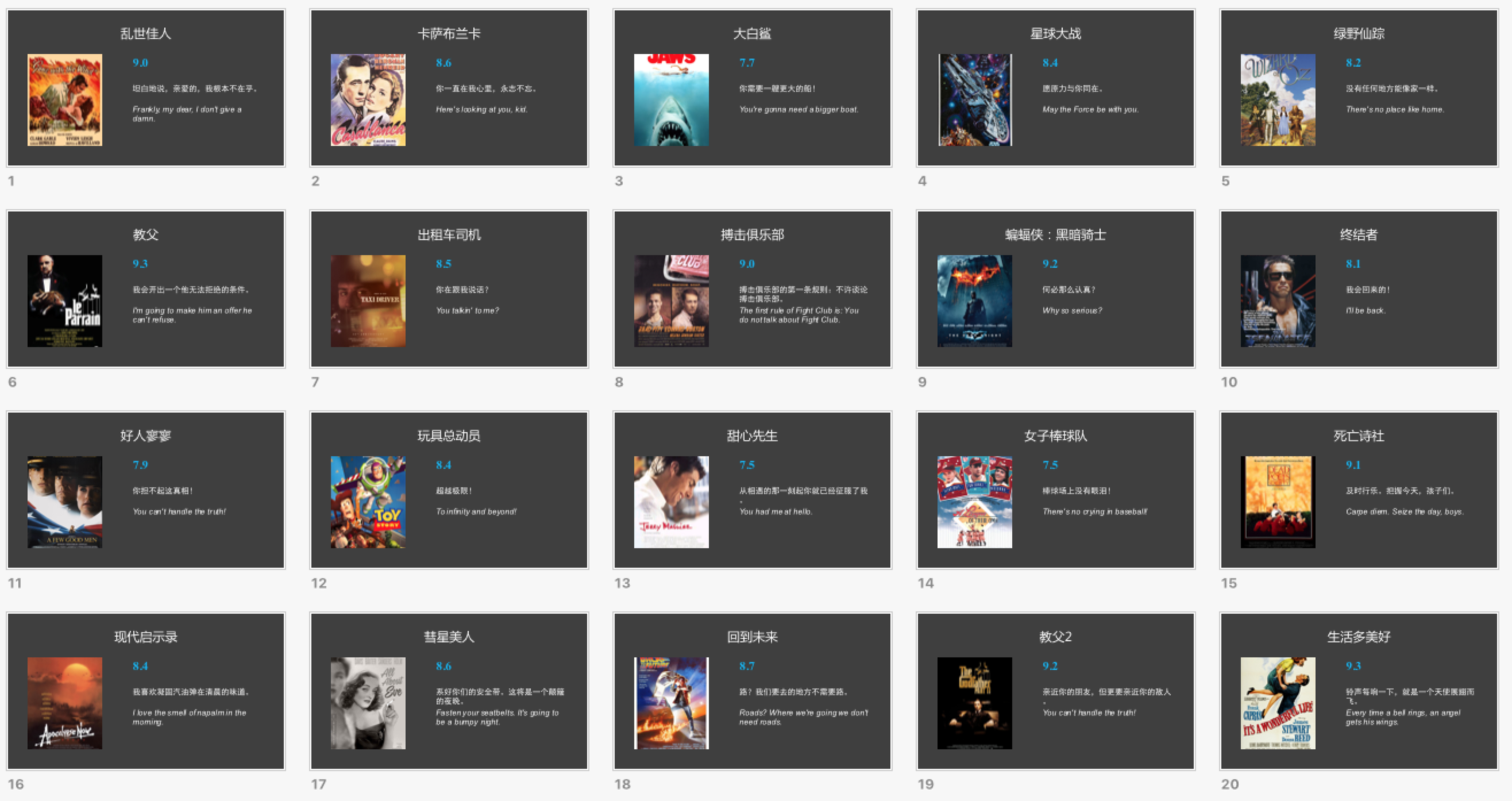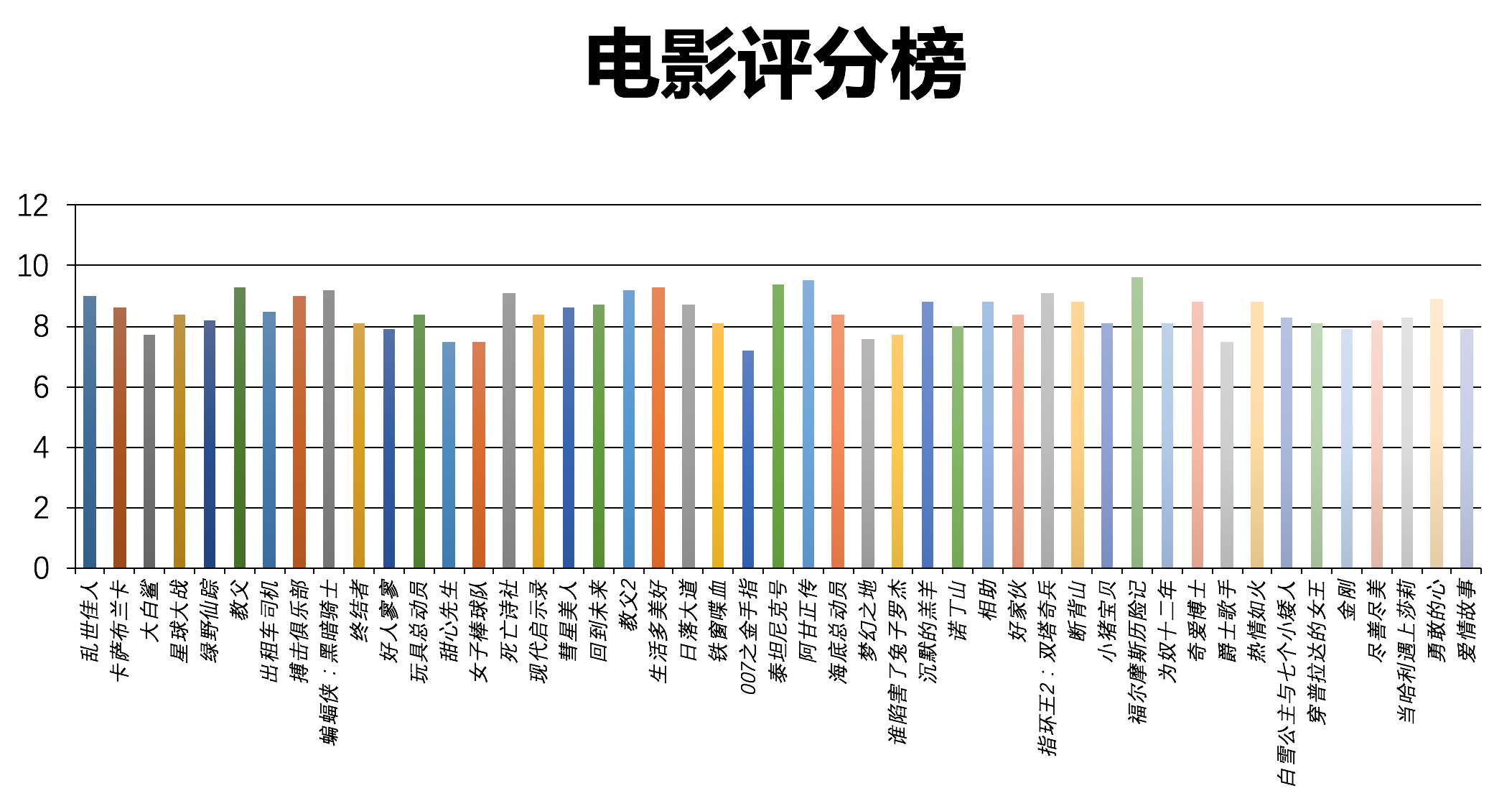# Python 处理 PPT 文件的实用姿势# ﻿

﻿

﻿

• 用PPT批量生成一些发布会风格的图片

• 从大量PPT中提取表格数据

• 用PPT批量制作简单动画

﻿

• pywin32，调用操作系统上已装好的PPT软件。

• python-pptx，根据OpenXML规范解析XML文件。

﻿

﻿

﻿

## 基本使用

﻿

PPT中基本元素包括：

• slide（幻灯片）：一个PPT由一系列slide构成。

• slide_master（幻灯片母版）：母版可定义主题样式基准。

• slide_layouts（模版）：创建幻灯片时可选择的模版。

• shape（形状）：包含一切可视元素，通过slide.shapes可访问slide内元素。

• placeholder（占位符）：在模板中占据位置，如图片、文字等。

• paragraph（段落）：文本段，可以直接设置整段文本样式。

• text（文本）：段落内的文本，通过paragraph.add_run()生成。

﻿

import pathlibfrom pptx import Presentationfrom pptx.enum.dml import MSO_THEME_COLORfrom pptx.util import Inches, Ptpath = list(pathlib.Path.cwd().parents).joinpath('data/automate/005ppt')out_path = path.joinpath('005ppt_basic.pptx')prs = Presentation()# 查看所有布局for lo in prs.slide_layouts:    print(lo.name)# 创建标题页title_slide_layout = prs.slide_layoutsprint(w, h, lo, ma)slide = prs.slides.add_slide(title_slide_layout)title = slide.shapes.titlesubtitle = slide.placeholderstitle.text = '只差一个程序员了'subtitle.text = '程一初'# 设置背景色fill = slide.shapes.fillfill.solid()fill.fore_color.theme_color = MSO_THEME_COLOR.ACCENT_1fill.fore_color.brightness = -0.25# 创建第一页内容bullet_slide_layout = prs.slide_layoutsslide = prs.slides.add_slide(bullet_slide_layout)shapes = slide.shapestitle_shape = shapes.titlebody_shape = shapes.placeholderstitle_shape.text = 'Python1024'tf = body_shape.text_frametf.text = '第一级内容'p = tf.add_paragraph()p.text = '第一级段落内容'p.level = 1p = tf.add_paragraph()p.text = '第二级段落内容'p.level = 2run = p.add_run()# 与python-docx里的用法类似，但内容设置在text中run.text = '自定义字体'run.font.name = '微软雅黑'run.font.size = Pt(25)run.font.bold = Trueprint(tf.paragraphs.text)# 从一个空白页开始创建内容blank_slide_layout = prs.slide_layoutsslide = prs.slides.add_slide(blank_slide_layout)left = top = width = height = Inches(1)txt_box = slide.shapes.add_textbox(left, top, width, height)tf = txt_box.text_frametf.text = '文本框内容'p = tf.add_paragraph()p.text = '文本框内增加一个粗体段落'p.font.bold = Truep = tf.add_paragraph()p.text = '文本框内增加一个大字体段落'p.font.size = Pt(40)prs.save(out_path)

﻿

## 图片和图形

﻿

PPT中图相关元素有3类：

• picture：图像，单位是Inch

• autoshape：形状，如流程图

• chart：图表，如直方图、饼图

﻿

### 生成流程图

﻿

import pathlibfrom pptx import Presentationfrom pptx.util import Inches, Ptfrom pptx.enum.shapes import MSO_SHAPEpath = list(pathlib.Path.cwd().parents).joinpath('data/automate/005ppt')out_path = path.joinpath('005ppt_image.pptx')img_path = path.joinpath('image.jpg')prs = Presentation()blank_slide_layout = prs.slide_layoutsslide = prs.slides.add_slide(blank_slide_layout)# 增加两张图片，一大一小left = top = Inches(1)pic = slide.shapes.add_picture(str(img_path), left, top)left = Inches(6)height = Inches(3)pic = slide.shapes.add_picture(str(img_path), left, top, height=height)# 增加一个AutoShape流程图title_only_slide_layout = prs.slide_layoutsslide = prs.slides.add_slide(title_only_slide_layout)shapes = slide.shapesshapes.title.text = '流程图'left = Inches(0.93)  # 0.93" centers this overall set of shapestop = Inches(3.0)width = Inches(1.75)height = Inches(1.0)shape = shapes.add_shape(MSO_SHAPE.PENTAGON, left, top, width, height)shape.text = '第一步'left = left + width - Inches(0.4)# 第一个形状左边平，第二个开始，左边有尖角，需要更长width = Inches(2.0)for n in range(2, 6):    shape = shapes.add_shape(MSO_SHAPE.CHEVRON, left, top, width, height)    shape.text = f'第{n}步'    left = left + width - Inches(0.4)prs.save(out_path)

﻿

### 生成图表

﻿

import pathlibfrom pptx import Presentationfrom pptx.util import Inches, Ptfrom pptx.chart.data import CategoryChartDatafrom pptx.enum.chart import XL_CHART_TYPE, XL_TICK_MARK, XL_LABEL_POSITION, XL_LEGEND_POSITIONfrom pptx.dml.color import RGBColorpath = list(pathlib.Path.cwd().parents).joinpath('data/automate/005ppt')out_path = path.joinpath('005ppt_chart.pptx')prs = Presentation()blank_slide_layout = prs.slide_layoutsslide = prs.slides.add_slide(blank_slide_layout)# 增加一个图表chart_data = CategoryChartData()chart_data.categories = ['A销售额', 'B销售额', 'C销售额']chart_data.add_series('Q1销售', (100, 120, 200))chart_data.add_series('Q2销售', (120, 150, 180))chart_data.add_series('Q3销售', (150, 180, 120))chart_data.add_series('Q4销售', (130, 210, 150))x, y, cx, cy = Inches(2), Inches(2), Inches(6), Inches(4)chart = slide.shapes.add_chart(    XL_CHART_TYPE.COLUMN_CLUSTERED, x, y, cx, cy, chart_data).chart# 设置图表的轴信息category_axis = chart.category_axiscategory_axis.has_major_gridlines = Truecategory_axis.minor_tick_mark = XL_TICK_MARK.OUTSIDEcategory_axis.tick_labels.font.italic = Truecategory_axis.tick_labels.font.size = Pt(24)plot = chart.plots # 用Plot对象访问标签plot.has_data_labels = Truedata_labels = plot.data_labelsdata_labels.font.size = Pt(13)data_labels.font.color.rgb = RGBColor(0x0A, 0x42, 0x80)data_labels.position = XL_LABEL_POSITION.INSIDE_ENDchart.has_legend = Truechart.legend.position = XL_LEGEND_POSITION.RIGHTchart.legend.include_in_layout = False# 增加一个饼图slide = prs.slides.add_slide(blank_slide_layout)chart_data = CategoryChartData()chart_data.categories = ['A销售额', 'B销售额', 'C销售额']chart_data.add_series('年度销售比', (0.276, 0.365, 0.359))chart = slide.shapes.add_chart(    XL_CHART_TYPE.PIE, x, y, cx, cy, chart_data).chartchart.has_legend = Truechart.legend.position = XL_LEGEND_POSITION.BOTTOMchart.legend.include_in_layout = Falsechart.plots.has_data_labels = Truedata_labels = chart.plots.data_labelsdata_labels.number_format = '0%'data_labels.position = XL_LABEL_POSITION.OUTSIDE_ENDprs.save(out_path)

﻿

• SmartArt，微软并没有公开发布过其标准格式，目前只有PowerPoint软件具备这个功能。

• PPT文件按页导出成图片，这也是PowerPoint软件功能，在Windows上可以借助win32com等模块调用PPT软件功能实现。

## 生成数据表格

﻿

PPT中也可以插入表格，只不过表格功能非常有限，仅用于展示。

import pathlibfrom pptx import Presentationfrom pptx.util import Inchespath = list(pathlib.Path.cwd().parents).joinpath('data/automate/005ppt')out_path = path.joinpath('005ppt_table.pptx')prs = Presentation()title_only_slide_layout = prs.slide_layoutsslide = prs.slides.add_slide(title_only_slide_layout)shapes = slide.shapesshapes.title.text = '表格数据'rows, cols = 5, 4left = top = Inches(2.0)width = Inches(6.0)height = Inches(0.8)table = shapes.add_table(rows, cols, left, top, width, height).tabledata = [    ('季度', 'A销售', 'B销售', 'C销售'), # 表头    ('Q1', 100, 120, 200),    ('Q2', 120, 150, 180),    ('Q3', 150, 180, 120),    ('Q4', 130, 210, 150),]for col in table.columns:    col.width = Inches(1.6)table.columns.width = Inches(1.0)for i, d in enumerate(data):    for j in range(4):        table.cell(i, j).text = str(data[i][j])prs.save(out_path)

﻿

## 导出素材和数据

﻿

### 导出图片和幻灯片备注

﻿

﻿

﻿

import pathlibfrom pptx import Presentationfrom pptx.util import Inches, Ptfrom pptx.enum.shapes import MSO_SHAPE_TYPE# 借用openpyxl的单位转换from openpyxl.utils.units import EMU_to_pixels as e2ppath = list(pathlib.Path.cwd().parents).joinpath('data/automate/005ppt')img_out_path = path.joinpath('005ppt_out_images')txt_out_path = path.joinpath('005ppt_notes.txt')in_path = path.joinpath('input_covid19.pptx')prs = Presentation(in_path)# EMU单位，1 Pixel = 9525 EMUw, h = prs.slide_width, prs.slide_heightprint(f'总共 {len(prs.slides)} 页，页宽 {e2p(w)} px，页高 {e2p(h)} px')cnt_img, txt_list = 0, []for i, slide in enumerate(prs.slides):    # 提取notes    notes_slide = slide.notes_slide    text_frame = notes_slide.notes_text_frame    txt_list.append(f'第 {i} 页:')    txt_list.append(text_frame.text)    # 提取图片    for s in slide.shapes:        if s.shape_type == MSO_SHAPE_TYPE.PICTURE:            img = s.image            cnt_img += 1            ext = img.content_type.split('/')            f_out_path = img_out_path.joinpath(f'{cnt_img}.{ext}')            with open(f_out_path, 'wb') as f:                f.write(img.blob)print(f'总导出 {cnt_img} 张图片.')with open(txt_out_path, 'w') as txt_fout:    txt_fout.write('\n'.join(txt_list))

﻿

### 导出表格和及图表中的数据

﻿

1. table的数据可以直接获取，但注意python-pptx中表格索引从0开始（openpyxl从1开始）

2. chart的数据在其内部，需要通过plots.categories提取

﻿

import pathlibfrom pptx import Presentationfrom pptx.chart.data import CategoryChartDatafrom pptx.util import Inches, Ptfrom pptx.enum.shapes import MSO_SHAPE_TYPEfrom openpyxl.utils.units import EMU_to_pixels as e2pfrom openpyxl import Workbookpath = list(pathlib.Path.cwd().parents).joinpath('data/automate/005ppt')table_out_path = path.joinpath('005ppt_out_tables.xlsx')chart_data_out_path = path.joinpath('005ppt_out_chart_data.xlsx')chart_out_path = path.joinpath('005ppt_out_charts')in_path = path.joinpath('input_table.pptx')prs = Presentation(in_path)# EMU单位，1 Pixel = 9525 EMUw, h = prs.slide_width, prs.slide_heightprint(f'总共 {len(prs.slides)} 页，页宽 {e2p(w)} px，页高 {e2p(h)} px')cnt_tables, cnt_charts = 0, 0xlsx_wb = Workbook()chart_wb = Workbook()for i, slide in enumerate(prs.slides, start=1):    for j, s in enumerate(slide.shapes, start=1):        if s.shape_type == MSO_SHAPE_TYPE.TABLE and s.has_table:            cnt_tables += 1            tbl = s.table            cnt_row, cnt_col = len(tbl.rows), len(tbl.columns)            print(f'增加表格 {cnt_tables}: {cnt_row} x {cnt_col}')            ws = xlsx_wb.create_sheet(f'{cnt_tables}_page_{i}')            for r in range(cnt_row):                for c in range(cnt_col):                    cell = tbl.cell(r,c)                    text_runs = []                    for paragraph in cell.text_frame.paragraphs:                        for run in paragraph.runs:                            text_runs.append(run.text)                    ws.cell(row=r+1, column=c+1, value=''.join(text_runs))        elif s.shape_type == MSO_SHAPE_TYPE.CHART:            chart = s.chart            cnt_charts += 1            # 导出Chart的数据到表格            data = chart.series            # for plot in chart.plots:            #     cat = list(plot.categories)            cat = list(chart.plots.categories) # 假设1个图表            if len(data) > 0:                ws = chart_wb.create_sheet(f'{cnt_charts}_page_{i}')                print(f'增加Chart数据 {cnt_charts}: {len(data)}行， {len(cat)} 列')                cat = ['标签'] + cat                ws.append(cat)                for d in data:                    values = [d.name] + list(d.values)                    ws.append(values)xlsx_wb.save(table_out_path)chart_wb.save(chart_data_out_path)

﻿

## 总结

﻿

﻿﻿﻿

﻿﻿

﻿﻿## 评论